# Year 5 Printable primary worksheets

All of our Year 5 resources come complete with answer sheets to save time for busy teachers and parents. Input your child’s scores and keep track of your child's progress.

Filters

### 573 results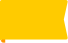New

### Creative writing - Friendship

What do you think makes a good friend? If your friend was sad, what would you do to make them feel better? Students answer questions about friendship.

Age: 9 - 10
1
2
3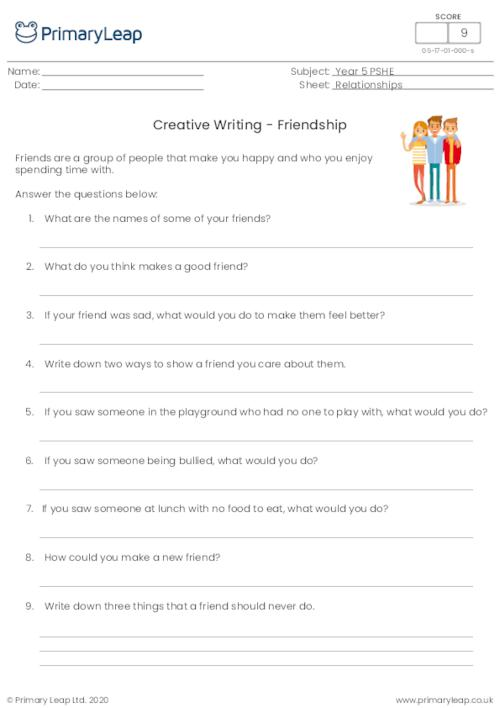New

### Design a Saving Water Poster

Students list ways they can save water at home and at school and design their own saving water poster.

Age: 9 - 10
1
2
3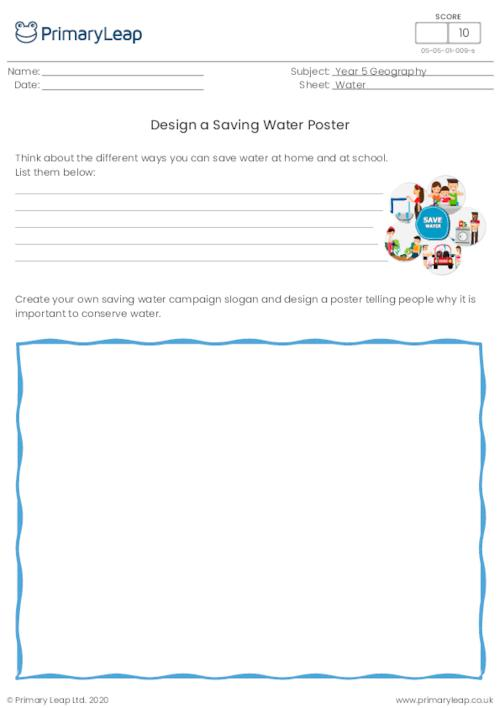New

### Translations

Translating s shape means sliding it up or down or side to side. You may not change the shape in any way. This worksheet asks students to translate each shape.

Age: 9 - 10
1
2
3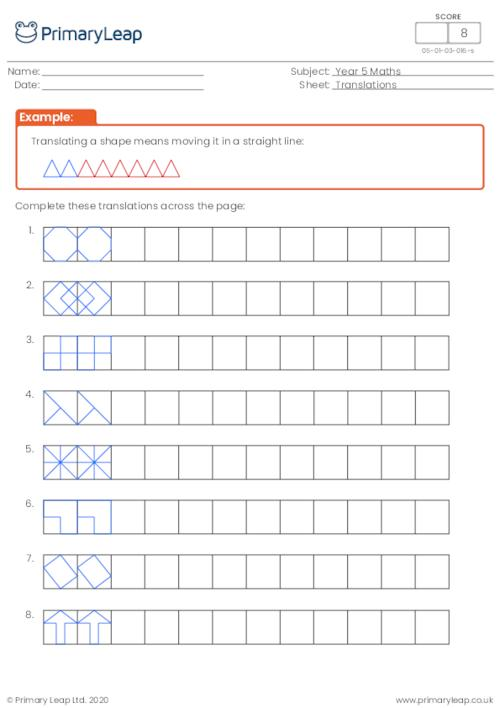New

### Properties of 3D shapes

This maths worksheet on 3D shapes asks students to complete the table by filling the missing properties.

Age: 9 - 10
1
2
3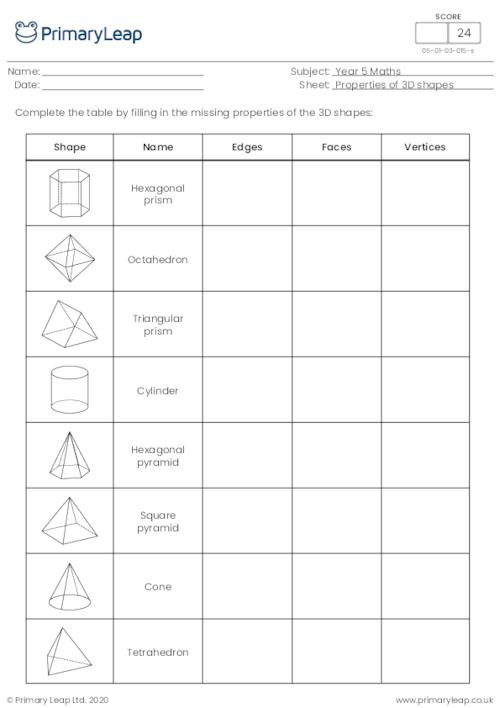New

### 3D shape nets

Students match the correct net with the 3D shapes by completing the table.

Age: 9 - 10
1
2
3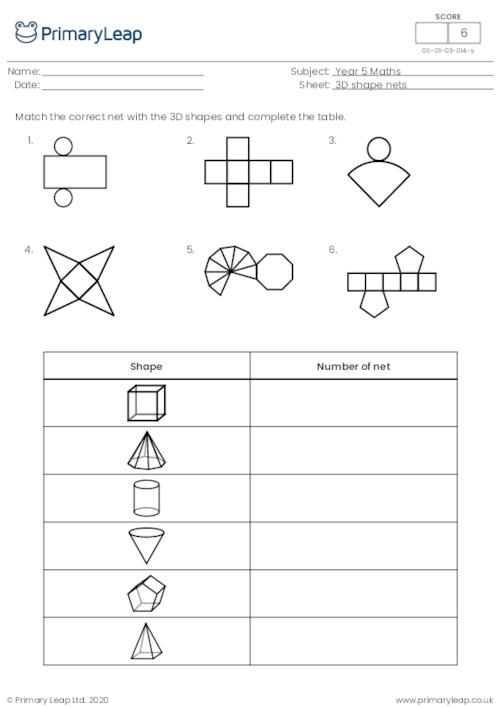New

### Naming 2D shapes

Students name the 2D shapes.

Age: 9 - 10
1
2
3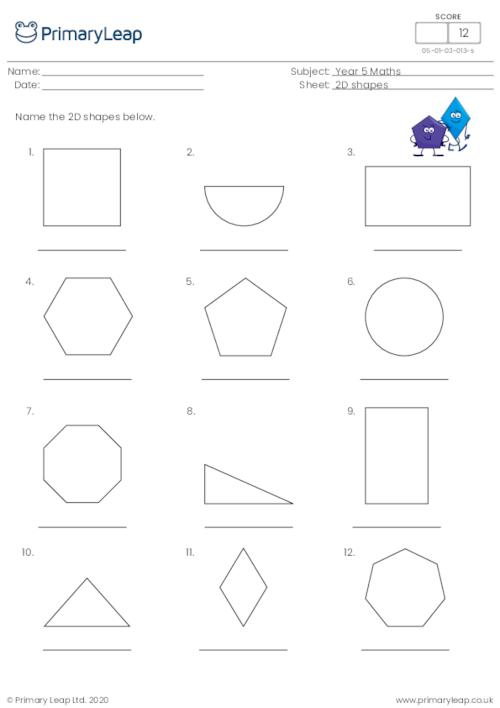New

### Classifying angles

Students label each angle with 'acute', 'obtuse' or 'reflex'.

Age: 9 - 10
1
2
3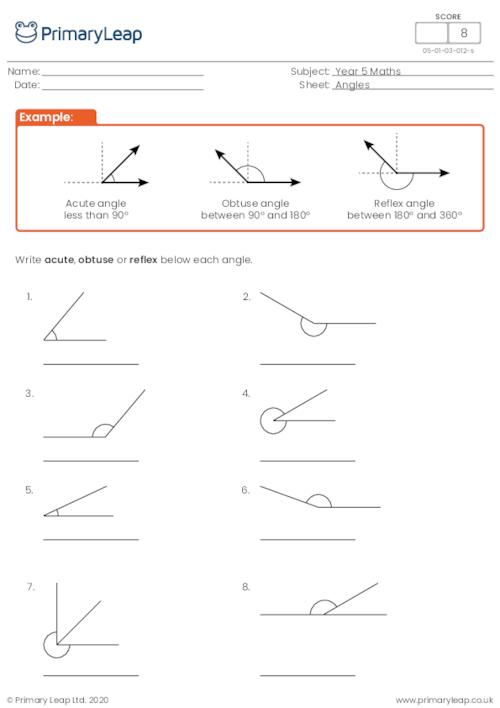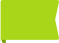Free

### Regular and irregular shapes

Students are asked to tick the correct box to show whether the shape is regular or irregular.

Age: 9 - 10
1
2
3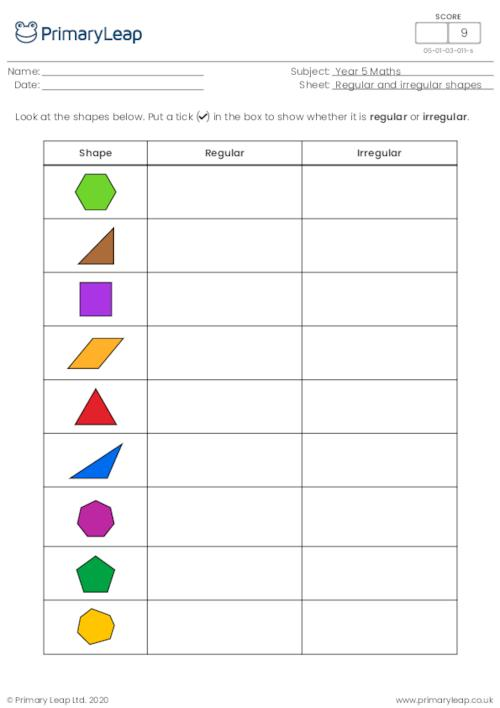New

### Estimating mass

Students estimate the correct weight of each object.

Age: 9 - 10
1
2
3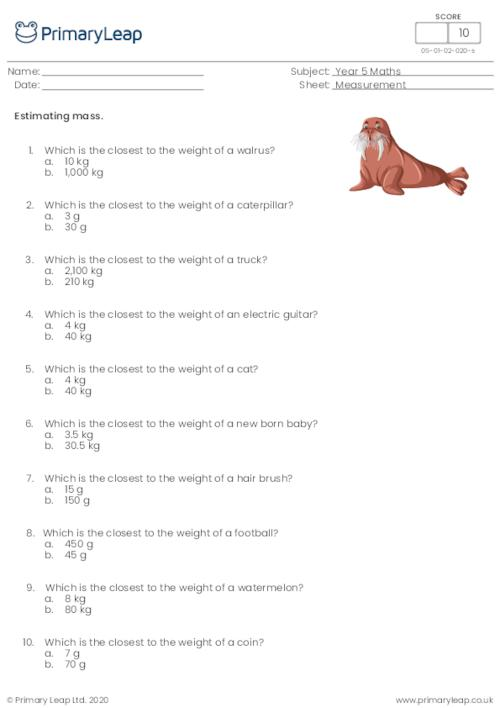New

### Metric units of weight

Students are asked to choose the correct unit to measure the weight of different objects.

Age: 9 - 10
1
2
3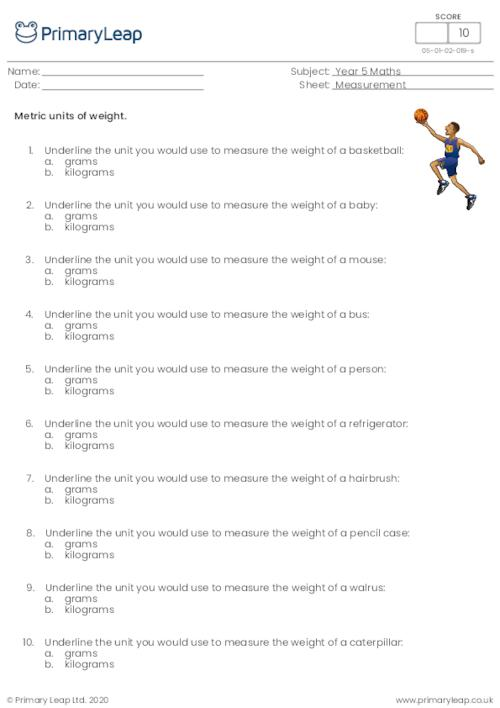New

### Converting units of length

Students read interesting facts and convert the different units of length.

Age: 9 - 10
1
2
3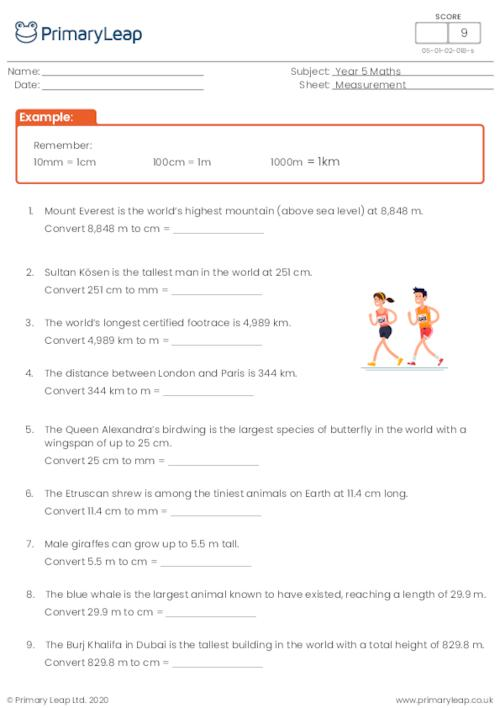New

### Metric units of length

Students are asked to choose the correct unit to measure different things.

Age: 9 - 10
1
2
3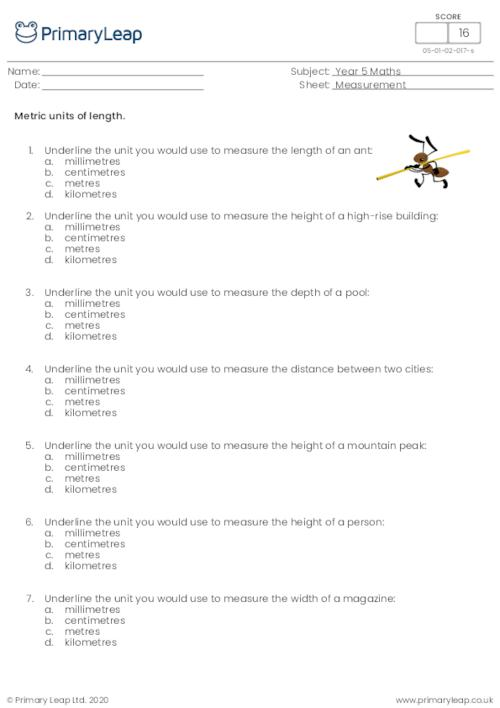New

### Area

Students complete the tables by filling in the missing measurements and then answer the questions.

Age: 9 - 10
1
2
3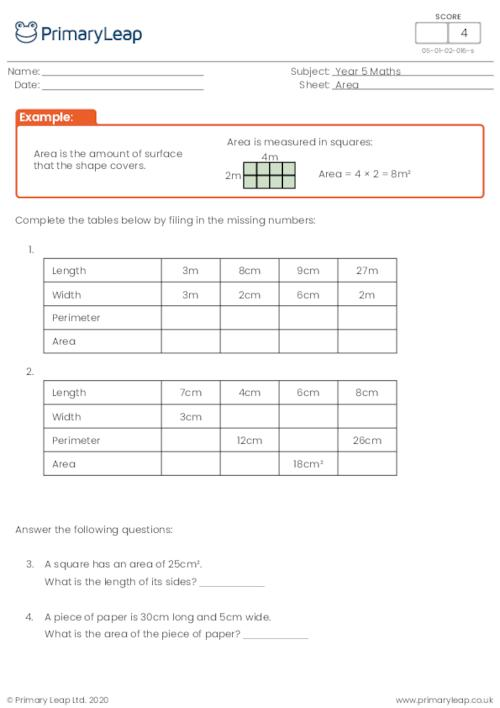### Finding the common factors of two numbers

Students are asked to find the common factors of two numbers.

Age: 9 - 10
1
2
3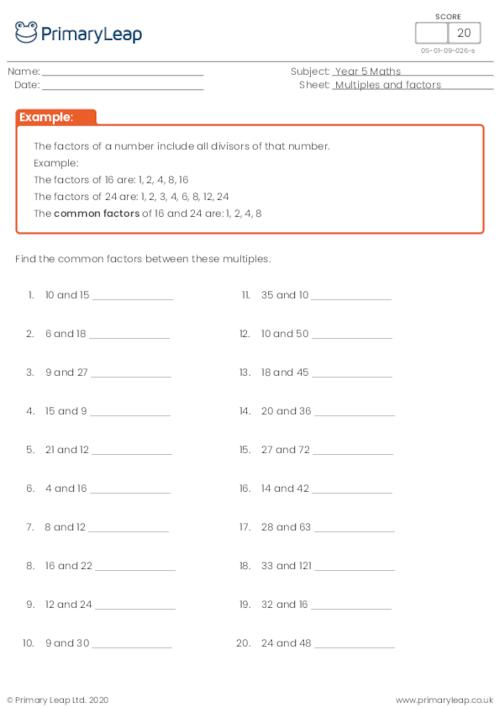### Finding the factor pairs of a number

Students are asked to find all the factor pairs of a number.

Age: 9 - 10
1
2
3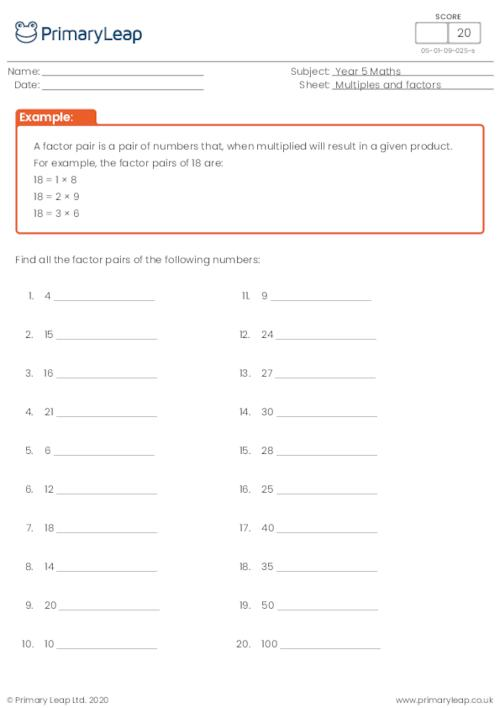### Identifying multiples and factors

Students identify the multiples and factors of each number sentence.

Age: 9 - 10
1
2
3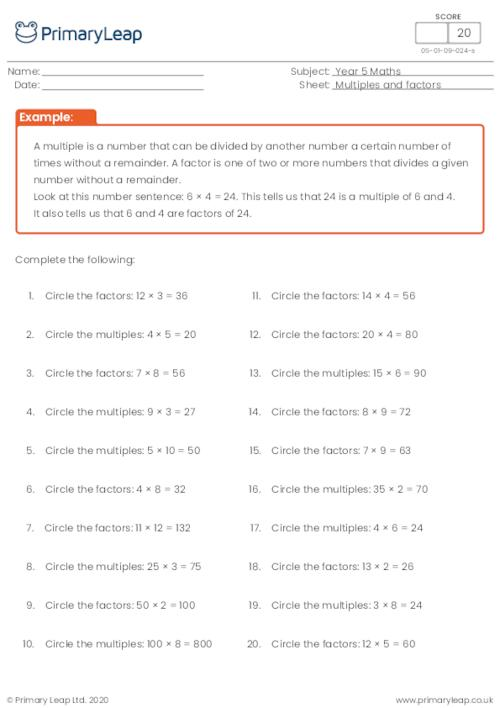### Recognising prime numbers up to 100

Students circle the prime numbers up to 100.

Age: 9 - 10
1
2
3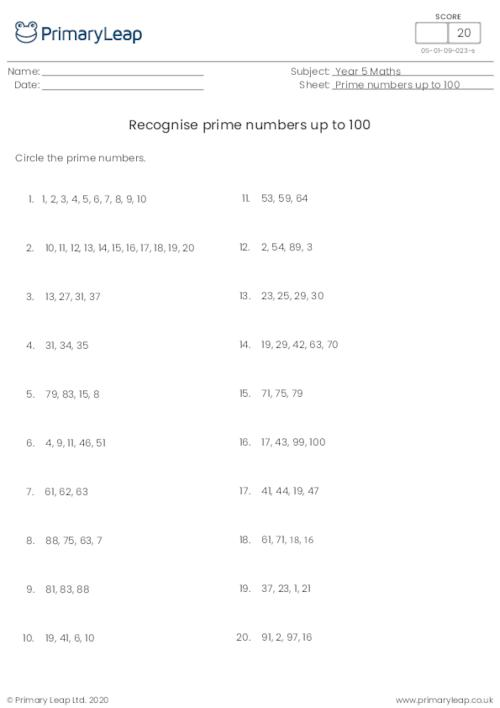### Writing prime numbers up to 20

Students practise writing the prime numbers up to 20.

Age: 9 - 10
1
2
3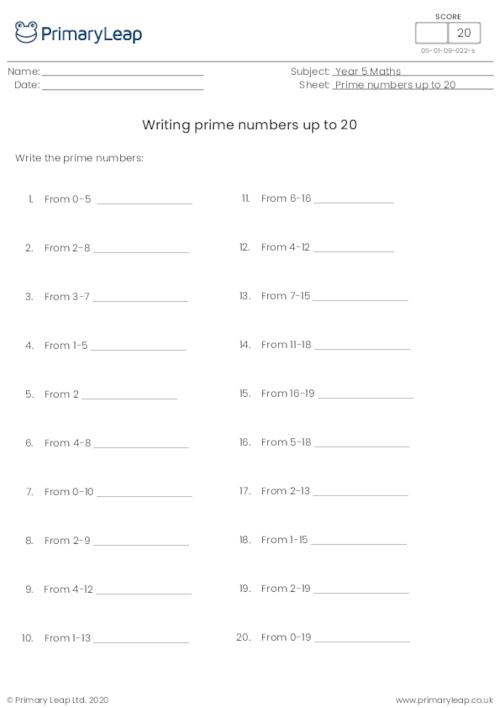### Recognising prime numbers up to 25

Students learn what a prime number is and recognise prime numbers up to 25.

Age: 9 - 10
1
2
3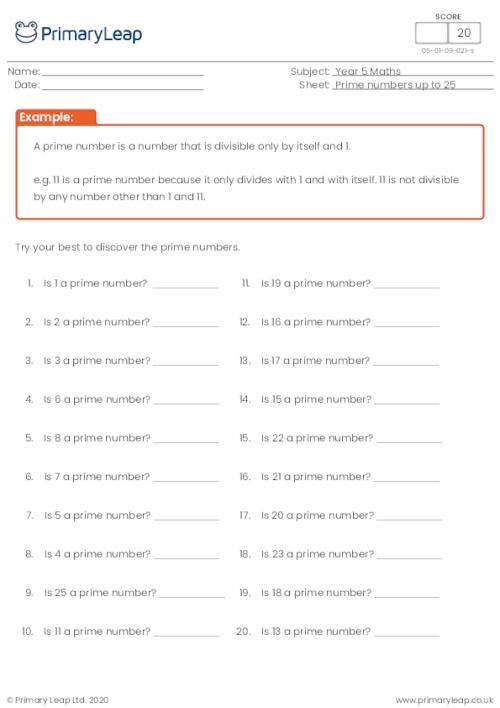### Recognising square numbers and cube numbers up to 12

Students practise finding the square of a number and the cube of a number without an example.

Age: 9 - 10
1
2
3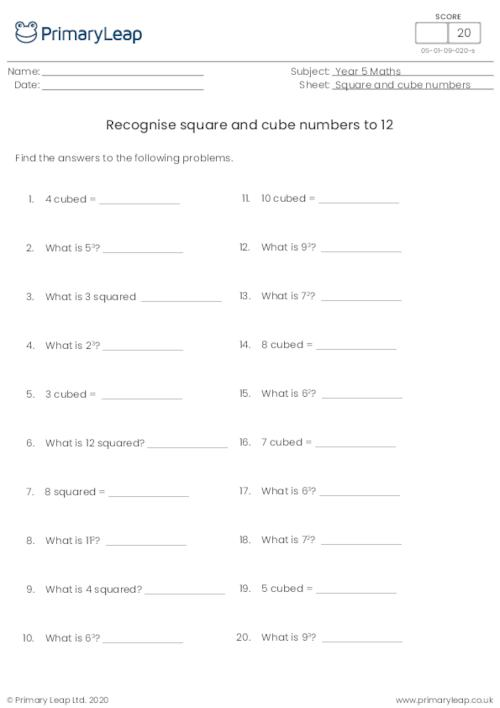### Recognising square numbers and cube numbers up to 12

Students practise finding the square of a number and the cube of a number.

Age: 9 - 10
1
2
3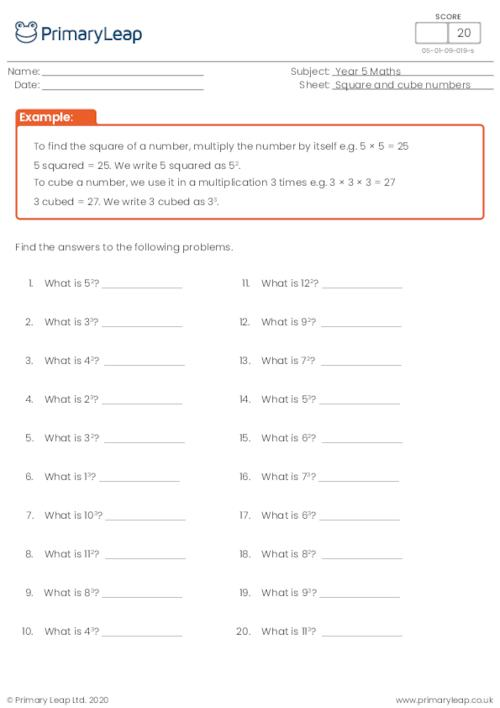### Recognising square numbers and cube numbers

Students learn how to square a number and how to cube a number.

Age: 9 - 10
1
2
3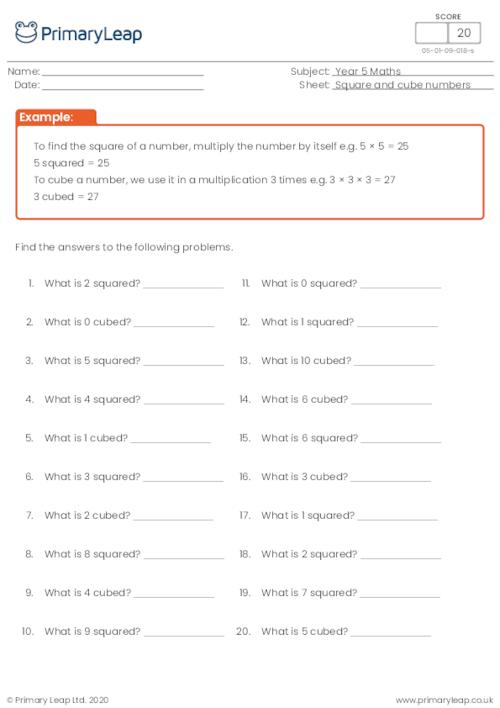### Multiply numbers up to 4 digits

Students are asked to multiply 4 digit numbers by a one or two-digit number.

Age: 9 - 10
1
2
3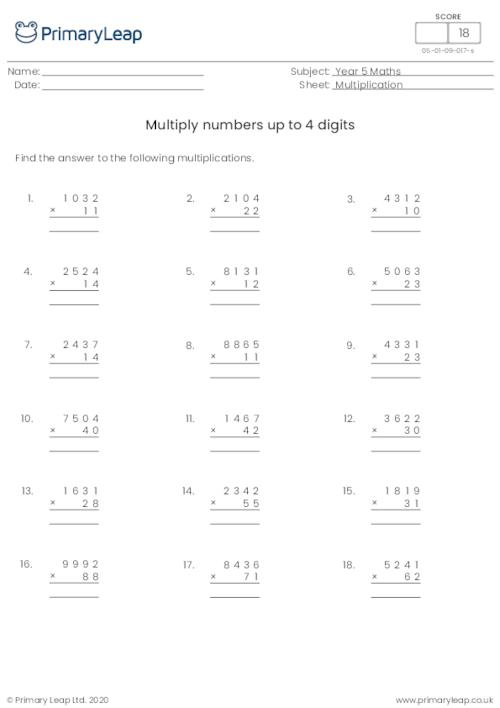### Multiply numbers up to 3 digits

Students practise multiplying 3 digit numbers by a 1 or 2 digit number.

Age: 9 - 10
1
2
3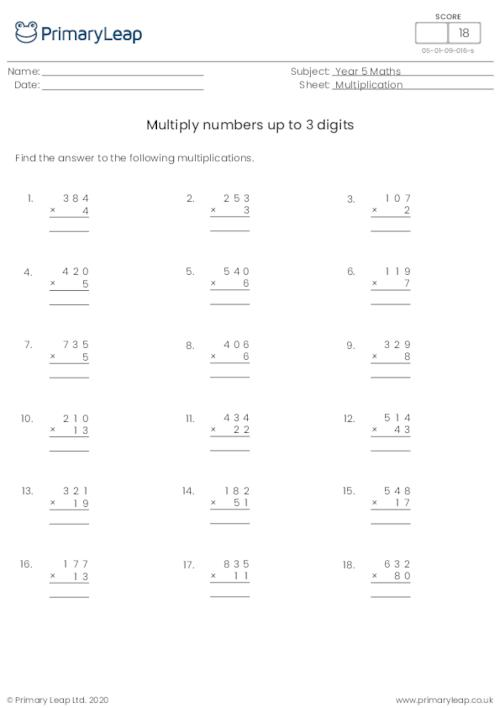### Multiply numbers up to 2 digits

Students practise multiplying numbers up to 2 digits.

Age: 9 - 10
1
2
3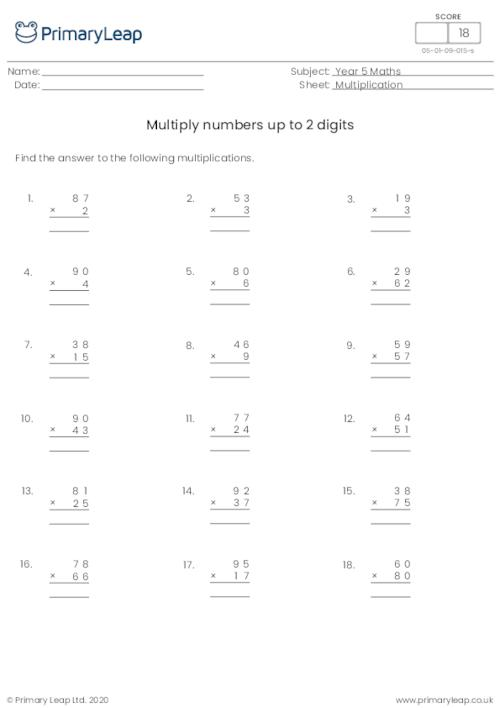### Multiply and divide decimals by 10, 100 and 1000

Students practise multiplying and dividing harder decimal numbers by 10, 100 and 1000.

Age: 9 - 10
1
2
3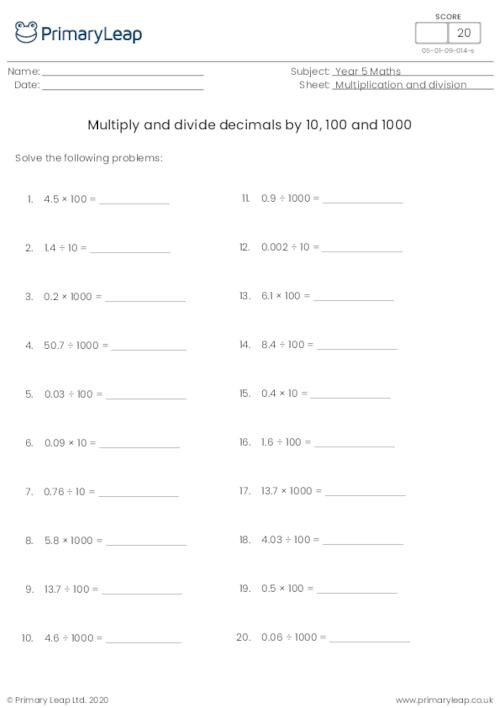### Multiply and divide decimals by 10, 100 and 1000

Students practise multiplying and dividing decimals by 10, 100 and 1000.

Age: 9 - 10
1
2
3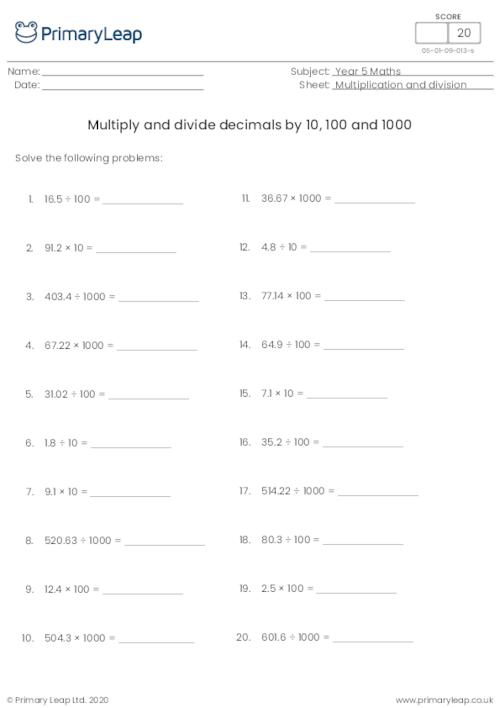### Multiply and divide decimals by 10, 100 and 1000

Students multiply and decimals by 10, 100 and 1000.

Age: 9 - 10
1
2
3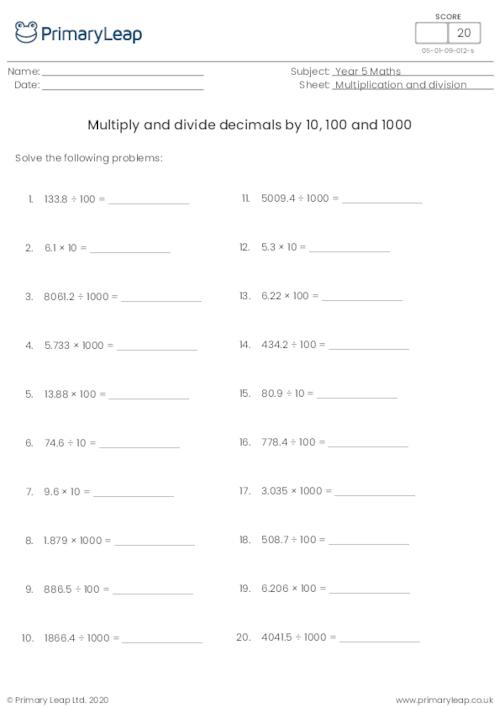### Dividing decimals by 10, 100 and 1000

Students practise dividing harder decimal numbers by 10, 100 and 1000.

Age: 9 - 10
1
2
3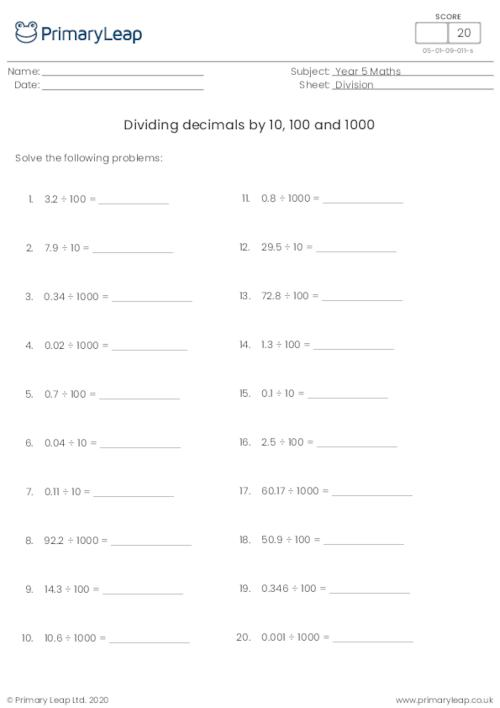### Dividing decimals by 10, 100 and 1000

Students practise dividing decimal numbers by 10, 100 and 1000.

Age: 9 - 10
1
2
3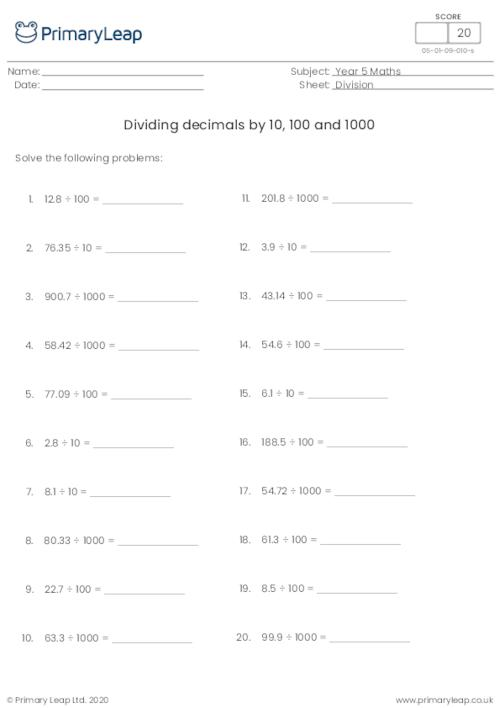### Dividing decimals by 10, 100 and 1000

Students practise dividing decimals by 10, 100 and 1000.

Age: 9 - 10
1
2
3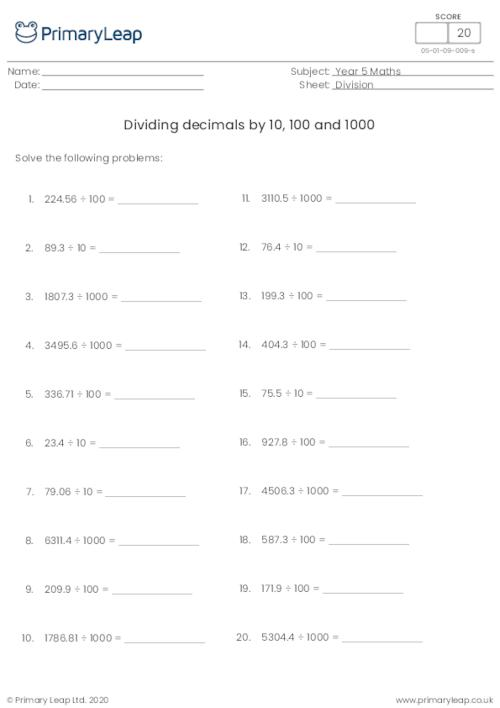### Dividing decimals by 1000

When dividing decimals by 1000, move the decimal point three spaces to the left. Students practise dividing decimals by 1000.

Age: 9 - 10
1
2
3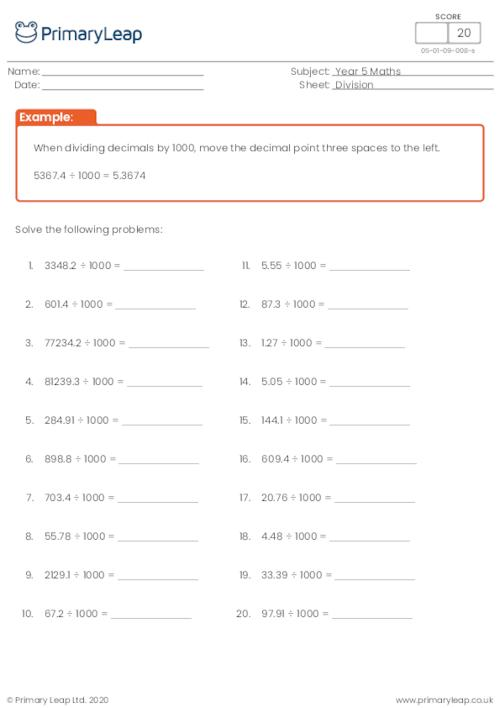### Dividing decimals by 100

When dividing decimals by 100, move the decimal point two spaces to the left. Students practise dividing decimals by 100.

Age: 9 - 10
1
2
3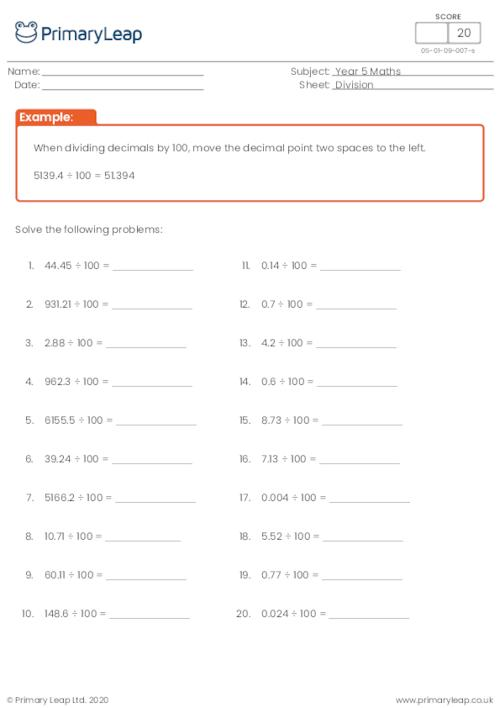### Dividing decimals by 10

When dividing decimals by 10, move the decimal point one space to the left. Students practise dividing decimal numbers by 10.

Age: 9 - 10
1
2
3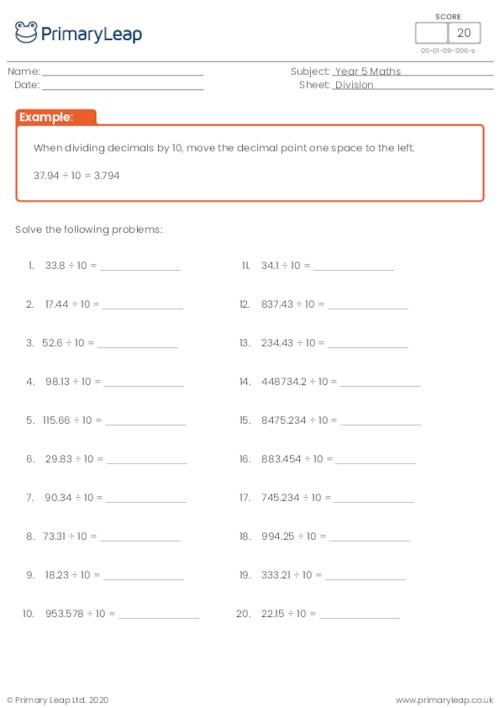### Multiply decimals by 10, 100 and 1000

Students practise multiplying decimals by 10, 100 and 1000 with harder numbers.

Age: 9 - 10
1
2
3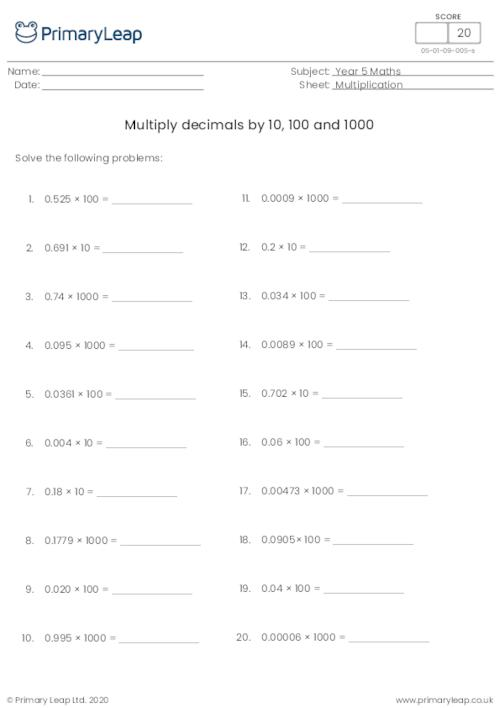### Multiply decimals by 10, 100 and 1000

This level 2 worksheet asks students to multiply the decimal numbers by 10, 100 and 1000.

Age: 9 - 10
1
2
3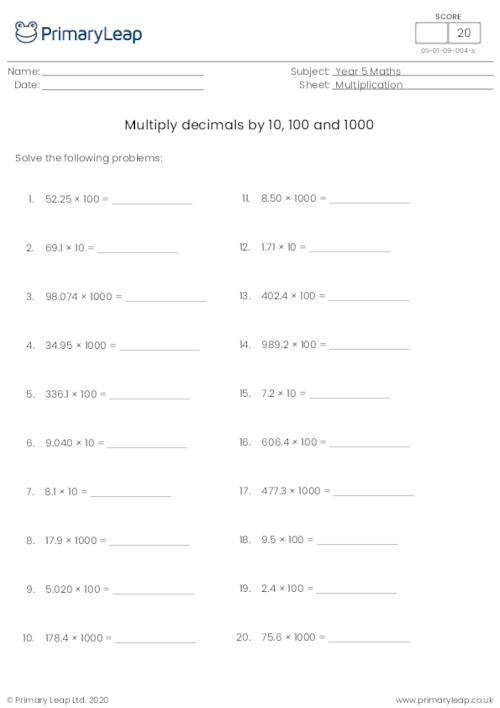### Multiply decimals by 10, 100 and 1000

Students practise multiplying decimals by 10, 100 and 1000.

Age: 9 - 10
1
2
3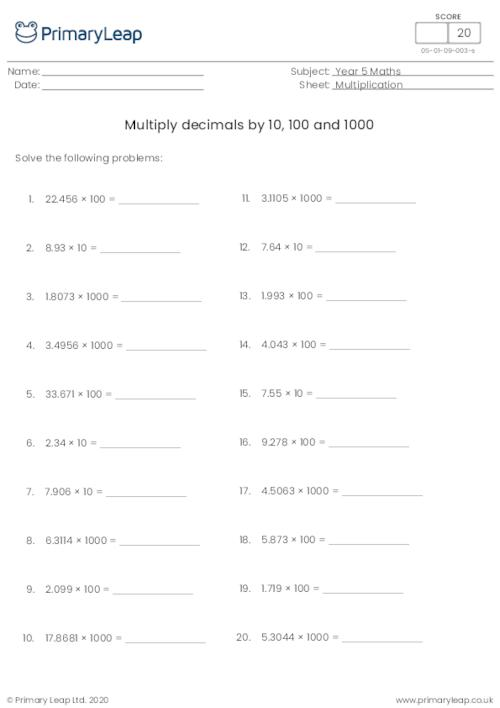### Multiply decimals by 1000

When multiplying decimals by 1000, move the decimal point three spaces to the right. Students practise multiplying decimals by 1000.

Age: 9 - 10
1
2
3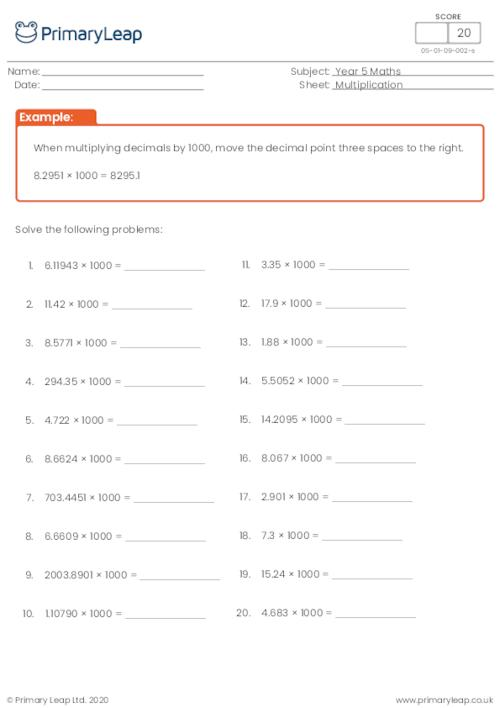### Multiply decimals by 100

When multiplying decimals by 100, move the decimal point two spaces to the right. Students practise multiplying decimal numbers by 100.

Age: 9 - 10
1
2
3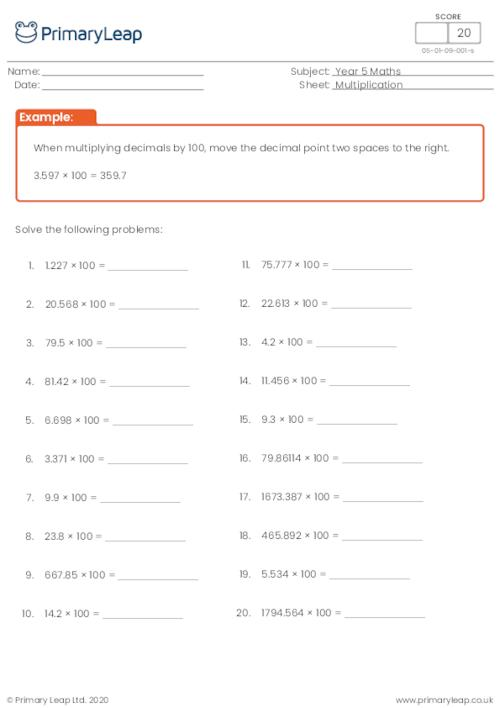### Multiply decimals by 10

When multiplying decimals by 10, move the decimal point one space to the right. Students are asked to multiply decimal numbers by 10.

Age: 9 - 10
1
2
3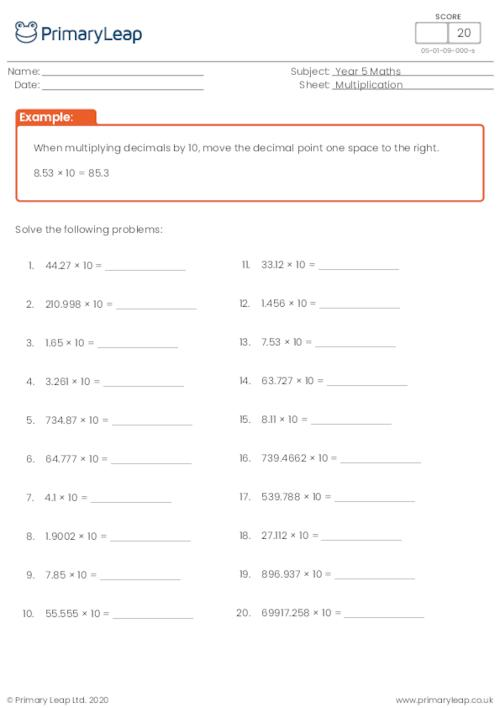### Converting decimals to fractions

Students convert 1 or 2 digit decimals to fractions.

Age: 9 - 10
1
2
3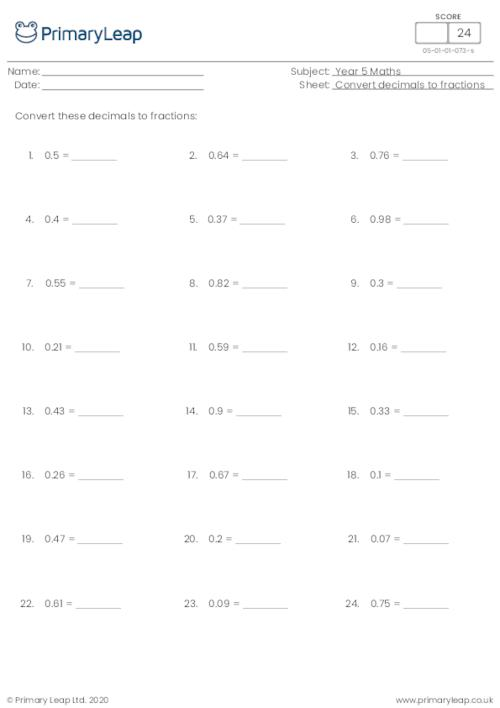### Converting fractions to decimals (denominator of 10 or 100)

Students practise converting fractions to decimals with a denominator of 10 or 100.

Age: 9 - 10
1
2
3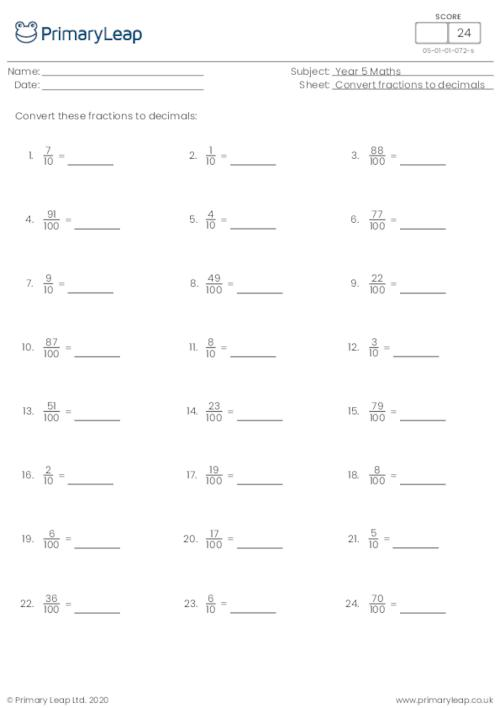### Converting fractions to decimals (denominator of 100)

Students practise converting fractions to decimals with a denominator of 100.

Age: 9 - 10
1
2
3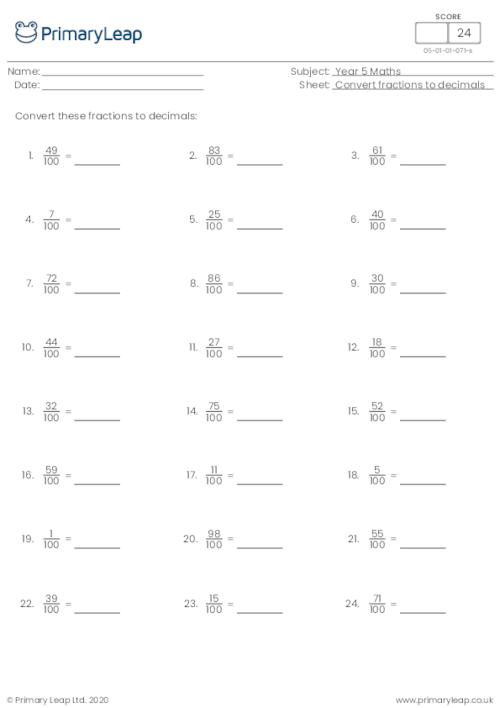### Subtracting fractions (same denominator)

This maths worksheet asks students to find the difference by subtracting the fractions.

Age: 9 - 10
1
2
3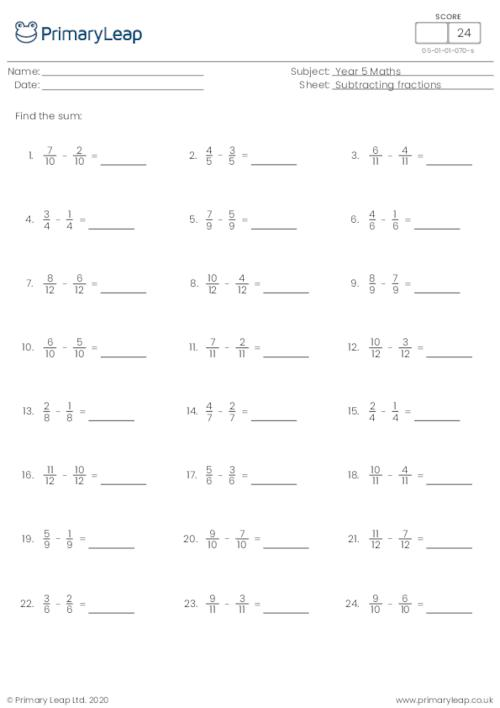This maths worksheet asks students to find the sum by adding the fractions.

Age: 9 - 10
1
2
3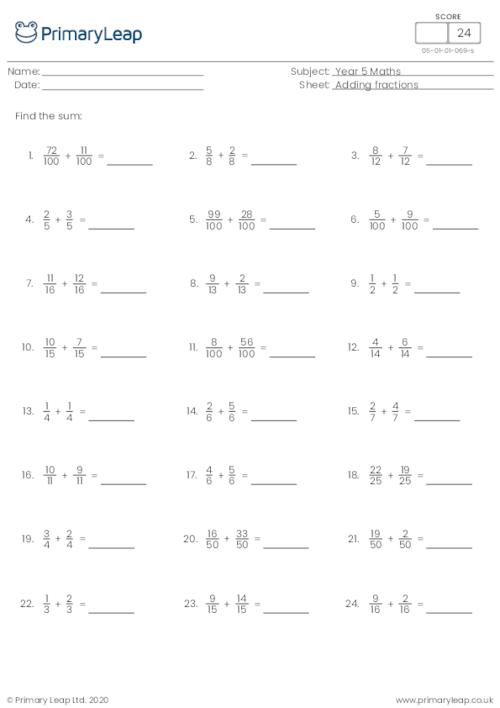### Adding 5 and 6-digit numbers in columns (with carrying)

This maths worksheet includes addition sums with two 5 and 6-digit numbers in columns. Students will practise carrying numbers.

Age: 9 - 10
1
2
3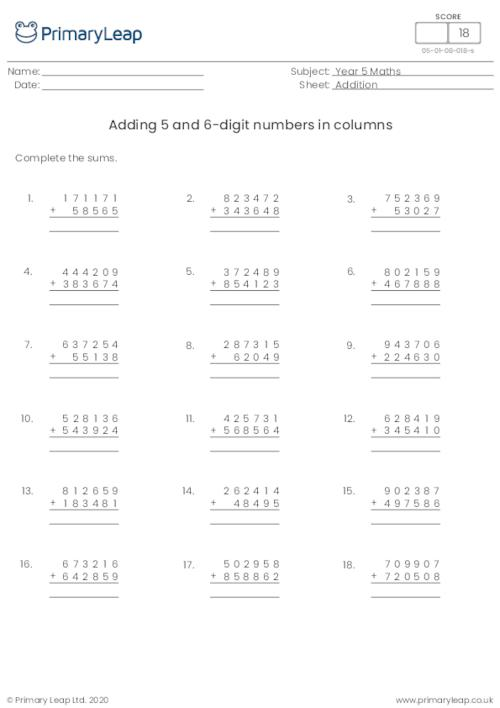### Adding 3-digit numbers in columns (with carrying)

This maths worksheet includes addition sums with two 3-digit numbers in columns. Students will practise carrying numbers.

Age: 9 - 10
1
2
3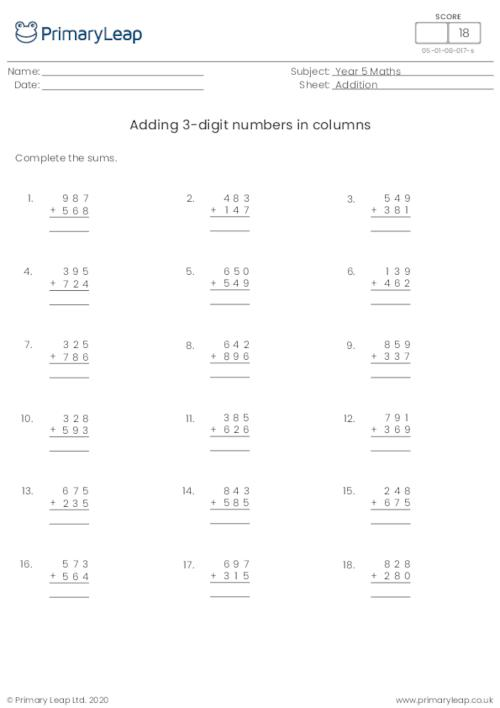### Adding 3-digit numbers in columns (with carrying)

This maths worksheet includes addition sums with two 3-digit numbers in columns. Students will practise carrying numbers.

Age: 9 - 10
1
2
3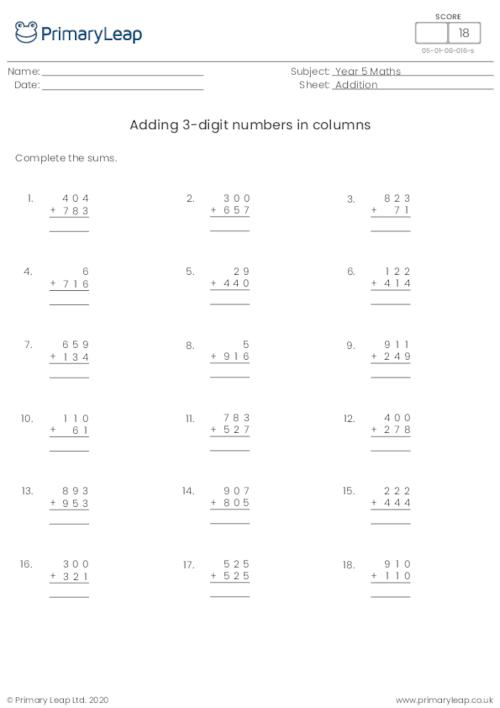of 12## Year 5 Worksheets - Created by teachers and loved by kids

Check out our ready-to-use Year 5 worksheets that can be used in the classroom or at home. Simply download or print and watch your child’s confidence grow with our easy to follow Year 5 printables.Learn

One of the best things about printable worksheets is that they make learning fun and easy. Explore our educational resources based on Year 5 and keep your children learning through these fun and engaging exercises.Progress

Our Year 5 printable worksheets are designed for progressive learning. Our engaging content enables a child to learn at their own speed and gain confidence quickly. When we help a child believe in themselves, we empower them for life.Improve